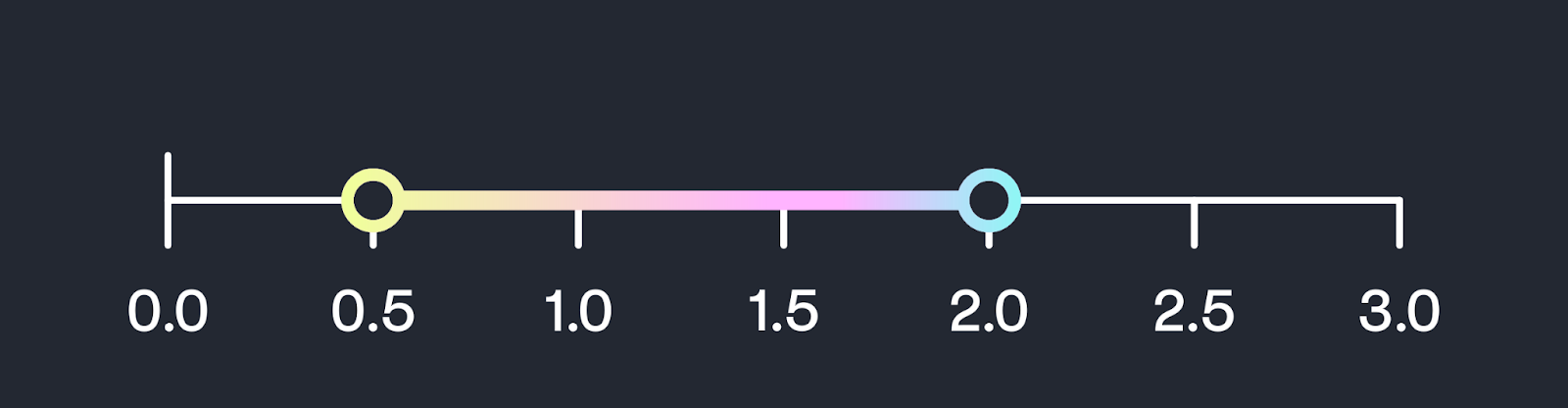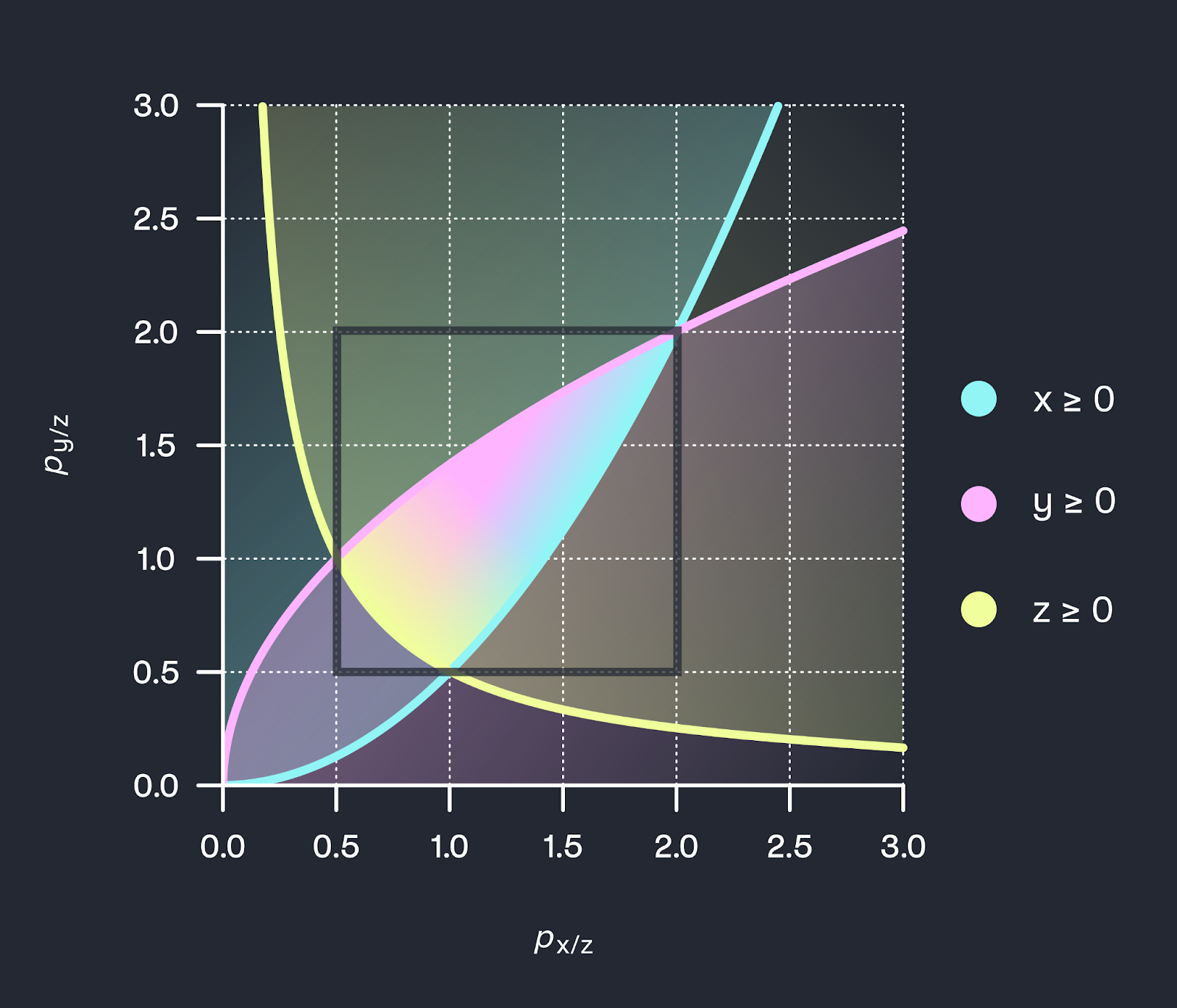# 3-CLPs

Cubic-Concentrated Liquidity Pools or 3-CLPs

## Description of 3-CLPs

Cubic CLPs, or 3-CLPs, concentrate the liquidity of three assets to a pricing range. The first 3-CLPs are designed for symmetric price ranges [α,1/α] on the three asset pairs in the pool. A given pool is parameterized by the pricing parameter α and the three assets that make up the pool .
Given quantities of real reserves (x,y,z) in the pool and the pool's pricing parameter α, the offset a can be calculated . This offset describes the amount the pool adds to real reserves to form the virtual reserves that achieve the pricing range.
Symmetric 3-CLPs use the following invariant: (x+a)(y+a)(z+a) = L^3
Like in any Balancer or Curve pool with more than two assets, the prices between different pairs of assets in the 3-CLP interact. For example, if asset x is traded against asset z, the pool’s spot price of asset y vs asset z is going to change. An implication of this is that the combinations of prices that are simultaneously offered by the pool at any point in time are constrained by a mathematical relationship.
Because of this relationship, understanding the multi-dimensional pricing bounds of the 3-CLP requires some thought. For the 2-CLP, the pricing bounds of the pool are very easy to understand: they are simply an interval of prices on a line, as shown below.stylistic visualization of 2-CLP price bounds
The graphic below visualizes the feasible pricing region of a 3-CLP, which is the region of spot prices that a 3-CLP may quote. Here we chose α=0.5, so that the pool does not price below 0.5 or above 2.0.stylistic visualization of the feasible pricing region of a 3-CLP
The feasible pricing region is parameterized by the prices of asset x and y, respectively, denoted in units of asset z. The third price pair, of x denoted in units of y, is the quotient of the two prices: px/y=px/z/py/z. In the figure, each included asset is represented with a different color.
The lines indicate price combinations where the respective reserve asset x, y, or z is exhausted in the pool, and the shaded regions indicate where the respective reserve asset has a positive balance in the pool.
The region where all colors overlap is the feasible pricing region - these are combinations of prices that the pool can quote. At the corner points, the pool only holds a single asset and two of the three asset pairs realize either the minimum or the maximum price bound (i.e., they are equal to α=0.5 or 1/α=2.0) . Further documentation is available here.

## Benefits of 3-CLPs

3-CLPs amplify the benefits of 2-CLPs:
• Capital efficiency: By concentrating liquidity between three assets, 3-CLPs achieve a 50% capital efficiency improvement from aggregating the third asset compared to a similar setup of trading pairs as 2-CLPs.
• Gas efficiency: Trading among three assets is more gas efficient than connecting two trades through two different 2-CLPs.
• User experience: 3-CLPs remain comparatively simple in architecture and user experience.Stylized representation of capital efficiency gains of 3-CLPs

## Risks of 3-CLPs

Using 3-CLPs also comes with certain risks, including the following. These risks include those of the 2-CLPs; we only explain these risks briefly. See the section on 2-CLPs for further details.
• Smart contract risk: the risk that an exploit or bug puts deposited assets at risk.
• Strategy risk: the risk that the portfolio strategy implied by the pool has worse payoffs than a different strategy (such as just holding the assets).
• Adverse selection risk: the risk that permanent price changes imply a loss to LPers because arbitrageurs take stale price quotes.
Compared to a 2-CLP, LPers in a 3-CLP are exposed to additional risks:
• Asset interaction risk: since the 3-CLP contains an additional asset, LPers are exposed to strategy and adverse selection risk due to two additional price pairs.
For example, if the price of one of the assets drops to a sufficiently low level, the pool will end up with only that least valuable asset (see the figure above). This phenomenon occurs in many multi-asset AMMs, such as the Curve stablecoin 3-pool. Just like strategy risk in the 2-pool, it can be reduced by choosing fundamentally connected assets and appropriate price bounds.
• Note that the pricing region of the 3-CLP is more complex than for other pools; this may mean that arbitrageurs need more time to get familiar with the functioning of the pool before it runs smoothly.

## Technical Specification

To read about the mathematical specification and implementation, see the below resources
 For technical reasons, the parameter provided to the smart contract is
$\sqrt{\alpha}$
$a = L \sqrt{\alpha}$